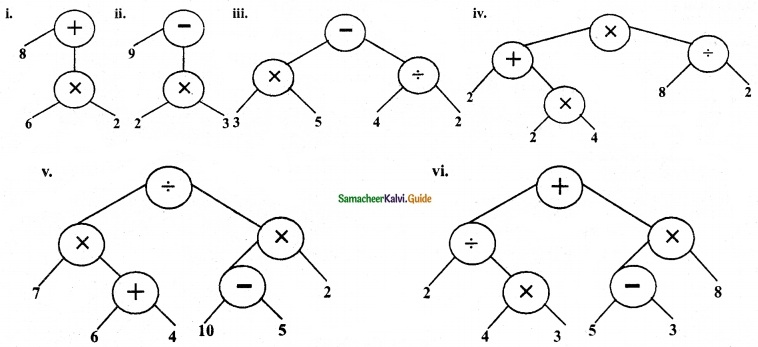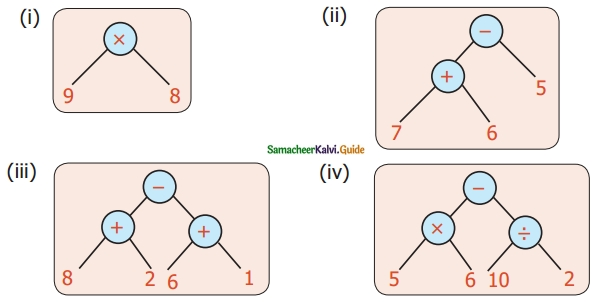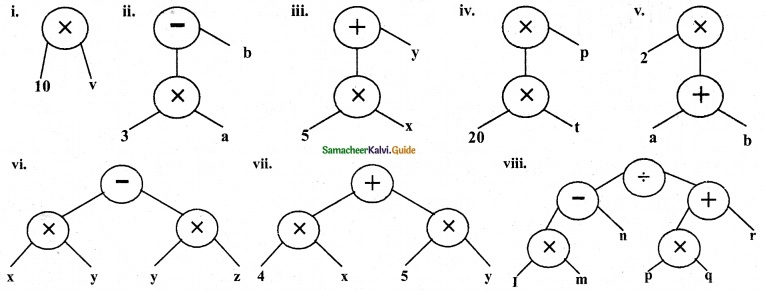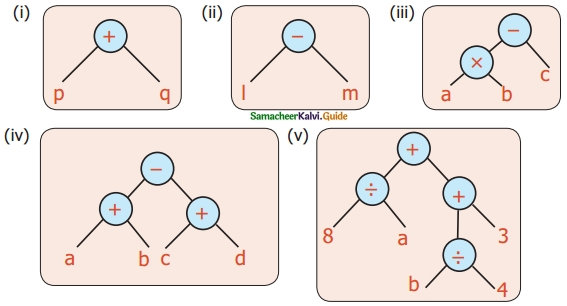Students can download Maths Chapter 5 Information Processing Ex 5.1 Questions and Answers, Notes, Samacheer Kalvi 6th Maths Guide Pdf helps you to revise the complete Tamilnadu State Board New Syllabus, helps students complete homework assignments and to score high marks in board exams.

## Tamilnadu Samacheer Kalvi 6th Maths Solutions Term 2 Chapter 5 Information Processing Ex 5.1

Question 1.
Convert the following numerical expressions into Tree diagrams
(i) 8 + (6 × 2)
(ii) 9 – (2 × 3)
(iii) (3 × 5) – (4 – 2)
(iv) [(2 × 4) + 2] × (8 – 2)]
(v) [(6 + 4) × 7] – [2 × (10 – 5)]
(vi) [(4 × 3) – 2] + [8 × (5 – 3)]
Solution:Question 2.
Convert the following tree diagrams into numerical expressions.Solution:
(i) The numerical Expression is 9 × 8
(ii) The numerical expression is (7 + 6) – 5
(iii) The numerical expression is (8 + 2) – (6 + 1)
(iv) The numerical expression is (5 × 6) – (10 ÷ 2)Question 3.
Convert the following algebraic expressions into tree diagrams.
(i) 10 v
(ii) 3a – b
(iii) 5x + y
(iv) 20t × p
(v) 2(a + b)
(vi) (x × y) – (y × z)
(vii) 4x + 5y
(viii) (Im – n) ÷ (pq + r)
Solution:Question 4.
Convert Tree diagrams into Algebraic expressions.Solution:
(i) Algebraic Expression is p + q
(ii) Algebraic Expression is l – m
(iii) Algebraic Expression is (a × b) – c (or) (ab) – c
(iv) Algebraic Expression is (a + b) – (c + d)
(v) Algebraic Expression is (8 ÷ a) + [ (6 ÷ 4) + 3]